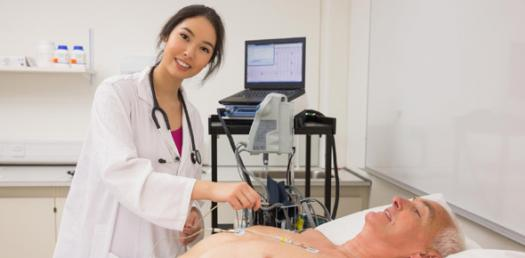# Stem 9 Practice Final

67 Questions | Attempts: 119
ShareSettingsSit back, relax and have fun with this Physical Science Practice quiz.

• 1.
Which of the following units is an SI base unit?
• A.

Centimeter

• B.

Kilogram

• C.

Cubic meter

• D.

Liter

• 2.
In a controlled experiment,
• A.

The outcome is controlled.

• B.

Results are obtained by computer models.

• C.

One variable is fixed while all others are changed.

• D.

One variable is changed while all others remain fixed.

• 3.
Matter is
• A.

Any visible solid that has mass.

• B.

Any liquid that takes up space and has mass.

• C.

Anything that takes up space and has mass.

• D.

Any liquid or solid that takes up space.

• 4.
What i the chemical formula for iron (III) oxide?
• A.

Fe2+

• B.

NaCl

• C.

I2

• D.

Fe2O3

• 5.
Which of the following is a mixture?
• A.

Air

• B.

Salt

• C.

Water

• D.

Sulfur

• 6.
Compounds and elements are
• A.

Always solids.

• B.

Mixtures.

• C.

Pure substances.

• D.

Dense.

• 7.
Which of the following is a physical change?
• A.

Melting ice cubes

• B.

Burning paper

• C.

Rusting iron

• D.

Burning gasoline

• 8.
If you add oil to water and shake the two liquids together, you will form a
• A.

Heterogeneous mixture.

• B.

Homogeneous mixture.

• C.

Miscible liquid.

• D.

Pure substance.

• 9.
During which change of state do atoms or molecules become more ordered?
• A.

Boiling

• B.

Condensation

• C.

Melting

• D.

Sublimation

• 10.
Which of the following describes what happens as the temperature of a gas in a balloon increases?
• A.

The volume of the gas increases and the speed of the particles in increases.

• B.

The speed of the particles decreases.

• C.

The volume decreases.

• D.

The pressure decreases.

• 11.
Materials that can flow to fit their containers include
• A.

Gases.

• B.

Liquids.

• C.

Both gases and liquids.

• D.

Neither gases or liquids.

• 12.
Which statement is NOT true of Bohr's model of the atom?
• A.

The nucleus can be compared to the sun.

• B.

Electrons orbit the nucleus.

• C.

An electron's path is not known exactly.

• D.

Electrons exist in energy levels.

• 13.
According to the moderns model of the atom,
• A.

Moving electrons form an electron cloud.

• B.

Electrons and protons circle neutrons.

• C.

Neutrons have a positive charge.

• D.

The number of protons an atom has varies.

• 14.
If an atom has a mass of 11 amu and contains five electrons, it's atomic number must be
• A.

55.

• B.

16.

• C.

6.

• D.

5.

• 15.
Which statement about atoms of elements in the same group of the periodic table is true?
• A.

They have the same number of protons.

• B.

They have the same mass number.

• C.

They have similar chemical properties.

• D.

They have the same number of total electrons.

• 16.
The majority of elements in the periodic table are
• A.

Nonmetals.

• B.

Conductors.

• C.

Synthetic.

• D.

Noble gases.

• 17.
An atom of which of the following elements is unlikely to form a positively charged ion?
• A.

Potassium, K

• B.

Selenium, Se

• C.

Barium, Ba

• D.

Silver, Ag

• 18.
Which of the following statements about krypton is NOT true?
• A.

Its molar mass is 83.79 g/mol Kr.

• B.

Its atomic number is 36.

• C.

One mole of krypton atoms has a mass of 41.90 g.

• D.

It is a noble gas.

• 19.
Compounds are different from mixtures because
• A.

Compounds are held together by chemical bonds.

• B.

Each substance in a compound maintains its own properties.

• C.

Each original substance in a compound remains chemically unchanged.

• D.

Mixtures are held together by chemical bonds.

• 20.
Which substance has ionic bonds?
• A.

CO

• B.

CO2

• C.

KCl

• D.

O2

• 21.
In an exothermic reaction,
• A.

Energy is conserved.

• B.

The formation of bonds in the product releases more energy than is required to break the bonds in the reactants.

• C.

Energy is released as bonds form.

• D.

All of the above.

• 22.
Which of the following is a homogeneous mixture?
• A.

• B.

Soil

• C.

Salt water

• D.

Vegetable soup

• 23.
A solution is _________ if it contains as much dissolved solute as it will hold under a given set of  conditions.
• A.

Saturated.

• B.

Dilute.

• C.

Supersaturated.

• D.

Concentrated

• 24.
Gases are more soluble in liquids when the pressure is __________ and the temperature is _________.
• A.

High, high

• B.

High, low

• C.

Low, high

• D.

Low, low

• 25.
Which ions does an acid form in solution?
• A.

Oxygen

• B.

Hydronium

• C.

Hydroxide

• D.

Sulfur

## Related TopicsBack to top
×

Wait!
Here's an interesting quiz for you.